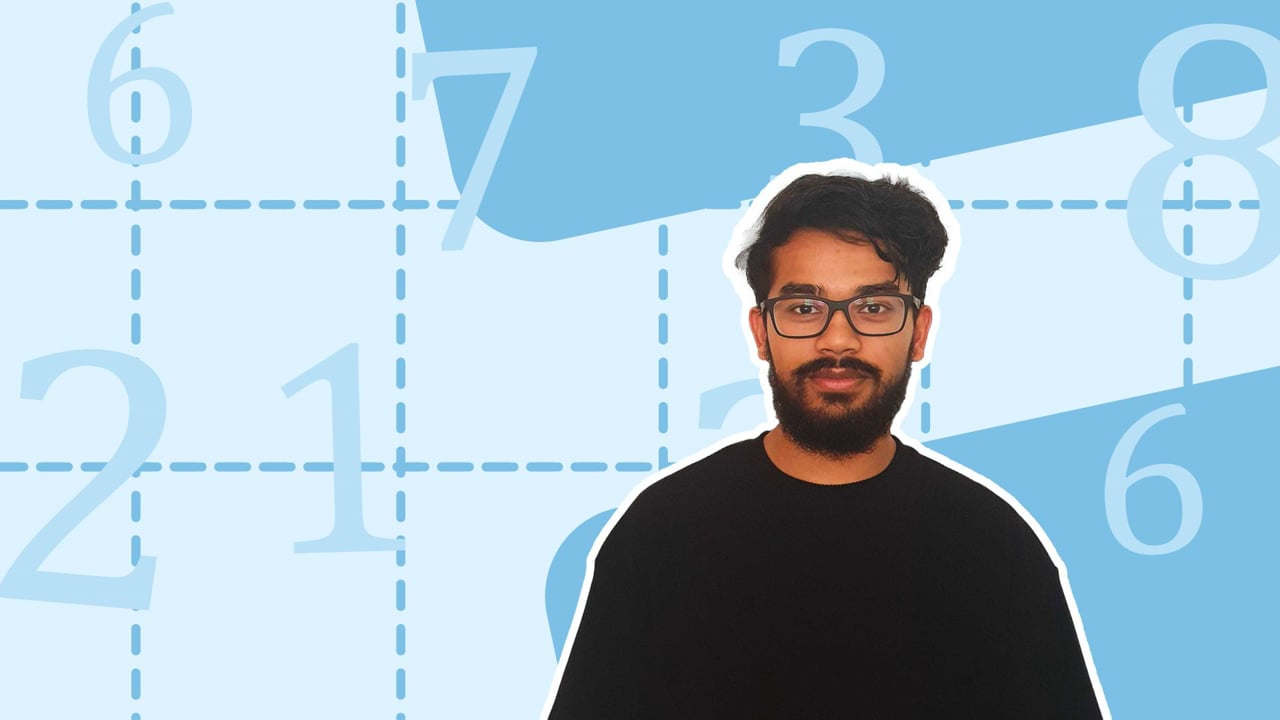Chapter overviewMaths

Number and place value

Multiplication and division

Fractions

Measurement

Geometry - properties of shapes

Geometry - position and direction

Statistics

Maths

# Mental maths strategies0%

Summary

# Mental maths strategies

## In a nutshell

Mental calculations can be performed faster by reorganising calculations into an easier format.

## Commutative property

The commutative property means that the order in which you multiply or add numbers will have no effect on the answer.

##### Example 1

Demonstrate the commutative law with $4 \times 3$.

Calculate $4 \times 3$.

$4\times 3 = 12$

Reorder the numbers to $3 \times4$.

$3 \times 4 =\underline{12}$​​

Note: The commutative property can be applied even if there are more than two numbers being multiplied together.

## Factor pairs

Factor pairs are two whole numbers which multiply to give the original number. Factor pairs can be used to break up a multiplication into easier steps.

##### Example 2

How many factor pairs does $12$ have?

Find all the whole numbers which multiply to give $12$.

{\begin{aligned} {12 \times1 = 12} \\ {6 \times 2=12}\\ {4 \times 3=12} \end{aligned}}

There are $\underline{3}$ factor pairs for $12$.

Note: The commutative property means the factor pairs can be written in the opposite order.

##### Example 3

Calculate $7 \times 16$.

$16$ can be split into $8$ and $2$. Use this to work out $7 \times 16$.

$7 \times 8 \times 2$

Multiply the numbers in an order you find easy.

\begin{aligned} 7\times 8 &= 56 \\ 56 \times 2 &= 112 \\ \\ &or \\ \\ 7 \times 2 &= 14 \\ 14 \times 8 &= 112 \end{aligned}​​

Therefore, $7 \times 16 = \underline{112}$

Note: $7$ is a prime number which means its only factor pair is $1$ and $7$.

FAQs

• Question: What are factor pairs?

Answer: Factor pairs are two whole numbers which multiply to give the original number.

• Question: What is the commutative property?

Answer: The commutative property means that the order in which numbers are multiplied has no effect on the answer.

Theory

Exercises